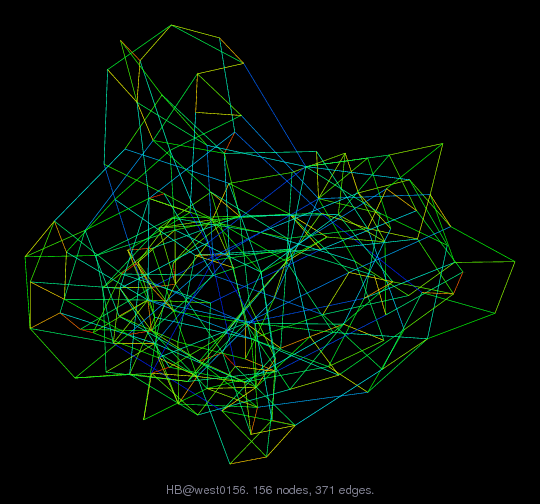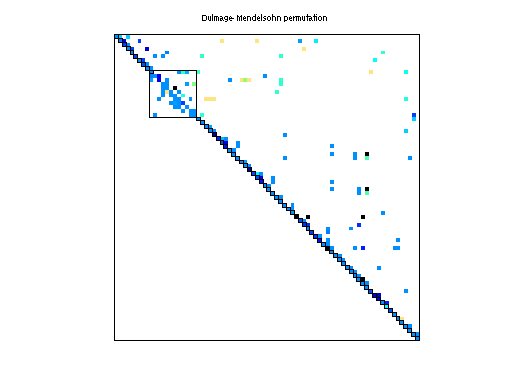Matrix: HB/west0156

Description: U SIMPLE CHEMICAL PLANT MODEL ( CHEM. ENG. FROM A. WESTERBERG )(bipartite graph drawing) (graph drawing of A+A')• Matrix group: HB
• download as a MATLAB mat-file, file size: 3 KB. Use UFget(264) or UFget('HB/west0156') in MATLAB.

 Matrix properties number of rows 156 number of columns 156 nonzeros 362 structural full rank? yes structural rank 156 # of blocks from dmperm 134 # strongly connected comp. 1 explicit zero entries 9 nonzero pattern symmetry 0% numeric value symmetry 0% type real structure unsymmetric Cholesky candidate? no positive definite? no

 author A. Westerberg editor I. Duff, R. Grimes, J. Lewis date 1983 kind chemical process simulation problem 2D/3D problem? no

 Ordering statistics: result nnz(chol(P*(A+A'+s*I)*P')) with AMD 1,944 Cholesky flop count 4.2e+04 nnz(L+U), no partial pivoting, with AMD 3,732 nnz(V) for QR, upper bound nnz(L) for LU, with COLAMD 348 nnz(R) for QR, upper bound nnz(U) for LU, with COLAMD 710

Note that all matrix statistics (except nonzero pattern symmetry) exclude the 9 explicit zero entries.

 SVD-based statistics: norm(A) 1.86737e+07 min(svd(A)) 8.10076e-12 cond(A) 2.30517e+18 rank(A) 154 sprank(A)-rank(A) 2 null space dimension 2 full numerical rank? no singular value gap 131189

 singular values (MAT file): click here SVD method used: s = svd (full (A)) ; status: ok